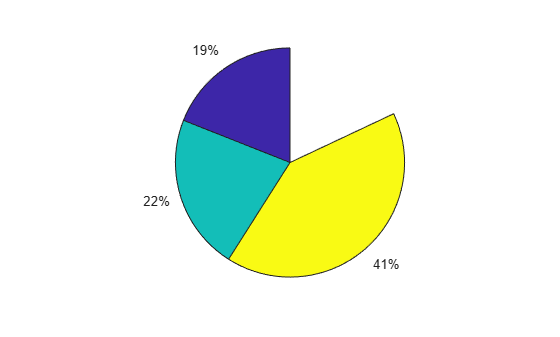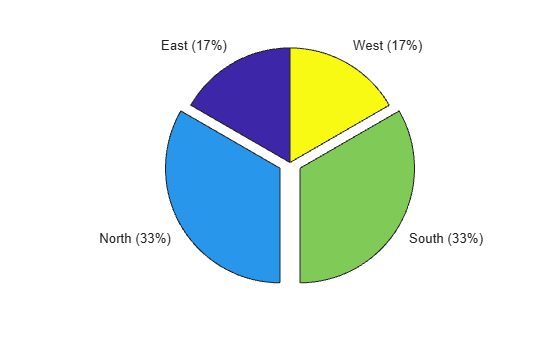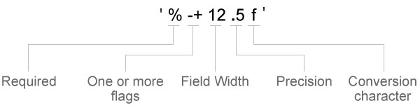# pie

•## Syntax

``pie(X)``
``pie(X,explode)``
``pie(X,labels)``
``pie(X,explode,labels)``
``pie(ax,___)``
``p = pie(___)``

## Description

example

````pie(X)` draws a pie chart using the data in `X`. Each slice of the pie chart represents an element in `X`.If `sum(X) ≤ 1`, then the values in `X` directly specify the areas of the pie slices. `pie` draws only a partial pie if ```sum(X) < 1```.If `sum(X) > 1`, then `pie` normalizes the values by `X/sum(X)` to determine the area of each slice of the pie.If `X` is of data type `categorical`, the slices correspond to categories. The area of each slice is the number of elements in the category divided by the number of elements in `X`.```

example

````pie(X,explode)` offsets slices from the pie. `explode` is a vector or matrix of zeros and nonzeros that correspond to `X`. The `pie` function offsets slices for the nonzero elements only in `explode`.If `X` is of data type `categorical`, then `explode` can be a vector of zeros and nonzeros corresponding to categories, or a cell array of the names of categories to offset.```

example

````pie(X,labels)` specifies options for labelling the pie slices. In this case, `X` must be numeric.```

example

````pie(X,explode,labels)` offsets slices and specifies the text labels. `X` can be numeric or categorical.```

example

````pie(ax,___)` plots into the axes specified by `ax` instead of into the current axes (`gca`). The option `ax` can precede any of the input argument combinations in the previous syntaxes.```

example

````p = pie(___)` returns a vector of patch and text graphics objects. The input can be any of the input argument combinations in the previous syntaxes.```

## Examples

collapse all

Create a pie chart of vector `X`.

```X = [1 3 0.5 2.5 2]; pie(X)```Offset the second and fourth pie slices by setting the corresponding `explode` elements to 1.

```explode = [0 1 0 1 0]; pie(X,explode)```Create a pie chart of vector `X` and label the slices.

```X = 1:3; labels = {'Taxes','Expenses','Profit'}; pie(X,labels)```Create a labeled pie chart, and then modify the color and font size of the text labels.

```X = 1:3; labels = {'Taxes','Expenses','Profit'}; p = pie(X,labels)``````p = 1x6 graphics array: Patch Text Patch Text Patch Text ```

Get the text object for the label 'Profit'. Change its color and font size. Use dot notation to set properties.

```t = p(6); t.BackgroundColor = 'cyan'; t.EdgeColor = 'red'; t.FontSize = 14;```Create a pie chart, and specify a format expression to display each label with three digits after the decimal point. To include a percent sign in the labels, specify `'%%'` at the end of the expression.

```X = [1/3 2/3]; pie(X,'%.3f%%')```Create a pie chart of vector `X` where the sum of the elements is less than 1.

```X = [0.19 0.22 0.41]; pie(X)````pie` draws a partial pie because the sum of the elements is less than 1.

Create vectors `y2010` and `y2011`, that contain financial data for two years. Then create a cell array containing the labels for the values.

```y2010 = [50 0 100 95]; y2011 = [65 22 97 120]; labels = {'Investments','Cash','Operations','Sales'};```

Create a 1-by-2 tiled chart layout, and display two pie charts that each have a title. Then display a shared legend in the east tile of the layout. To do this, call the `legend` function with a return argument to store the legend object. Then move the legend to the east tile by setting the `Layout.Tile` property to `'east'`.

```t = tiledlayout(1,2,'TileSpacing','compact'); % Create pie charts ax1 = nexttile; pie(ax1,y2010) title('2010') ax2 = nexttile; pie(ax2,y2011) title('2011') % Create legend lgd = legend(labels); lgd.Layout.Tile = 'east';```Plot a categorical pie chart with offset slices corresponding to categories.

```X = categorical({'North','South','North','East','South','West'}); explode = {'North','South'}; pie(X,explode)```Now, use a logical vector to offset the same slices.

```explode = [0 1 1 0]; pie(X,explode)```Plot a categorical pie chart without any offset slices and label the slices. When `X` is of data type `categorical` you must specify the input argument `explode`. To specify labels without any offset slices, specify `explode` as an empty cell array, and `labels` as the labels.

```X = categorical({'North','South','North','East','South','West'}); explode = {}; labels = {'E','N','S','W'}; pie(X,explode,labels)```Now, offset a slice and label all slices.

```X = categorical({'North','South','North','East','South','West'}); explode = {'West'}; labels = {'E','N','S','W'}; pie(X,explode,labels)```## Input Arguments

collapse all

Input vector or matrix.

• If `X` is numeric, then all values in `X` must be finite.

• If `X` is categorical, then `pie` ignores undefined elements.

Data Types: `double|categorical`

Offset slices, specified as a numeric vector or matrix, a cell array of character vectors, or a string array.

• If `X` is numeric, then `explode` must be a logical or numeric vector or matrix of zeros and nonzeros that correspond to `X`. A true (nonzero) value offsets the corresponding slice from the center of the pie chart, so that `X(i,j)` is offset from the center if `explode(i,j)` is nonzero. `explode` must be the same size as `X`.

• If `X` is categorical, then `explode` can be a cell array of character vectors that are category names or a string array of category names. `pie` offsets slices corresponding to categories in `explode`.

• If `X` is categorical, then `explode` also can be a logical or numeric vector with elements that correspond to each category in `X`. The `pie` function offsets slices corresponding to true (nonzero) in category order.

Label options, specified as an array of text labels or a format expression. Use this argument to display custom text labels with the pie slices, or to display the percentage values that MATLAB® provides in a specific format.

Note

To specify the label options when `X` contains categorical values, you must specify the `explode` argument before the label options.

#### Display Text Labels

Specify the labels as a cell array of character vectors or as a string array.

When `X` contains numeric values, the number of elements in `labels` must equal the number of elements in `X`.

When `X` contains categorical values, the number and order of the elements in `labels` must equal the number and order of the categories in `X`. To determine the number and order of the categories, use the `categories` function.

#### Display Percentages in a Specific Format

Specify a format expression as a character vector or as a string scalar. The format expression begins with a percent symbol (`%`) followed by a series of identifiers.All the identifiers are optional except for the conversion character. Specify the identifiers in this order:

1. One or more flags — Options for displaying plus signs, decimal points, and justifying the labels.

• Plus sign (`+`) — Display a plus sign next to positive values.

• Hash symbol (`#`) — Display the decimal point even when the precision is `0`, such as in `'50.'`.

• Minus sign (`–`) — Left justify the labels and pad the ends of the labels with spaces instead of padding them at the beginning.

2. Field width — Minimum number of characters to display in the label. Specify the field width as an integer value. If the number of digits in a label is smaller than the field width, then the label is padded with spaces.

3. Precision — Number of digits to the right of the decimal point. Specify the precision as a decimal point followed by an integer value.

4. Conversion character — Fixed-point or exponential notation. For a list of conversion characters, see the table below. If you specify a conversion character that does not fit the data, MATLAB uses `%e` instead.

Conversion CharacterDescriptionExample
`f`Fixed-point notation. The precision value indicates the number of decimal places.`'%.4f%%'` displays the label for a slice that is 6.25% of the pie as `6.2500%`.
`e`Exponential notation. The precision value indicates the number of decimal places.`'%.4e%%'` displays the label for a slice that is 6.25% of the pie as `6.2500e+00%`.
`g`The more compact version of `e` or `f`, with no trailing zeros. The precision value indicates the maximum number of decimal places.`'%.4g%%'`displays the label for a slice that is 6.25% of the pie as `6.25%`.

In addition to the identifiers listed above, you can also specify literal text at the beginning or end of the format expression. To display a single quotation mark, use `''`. To display a percent symbol, use `%%`. For example, `'%.2f%%'` displays the labels with two trailing decimal places followed by a percent symbol.

Axes object. Use `ax` to plot the pie chart in a specific axes instead of the current axes (`gca`).

## Output Arguments

collapse all

`Patch` and `Text` objects, returned as a vector. For more information, see Patch Properties and Text Properties.

## Version History

Introduced before R2006a

expand all

Behavior changed in R2019b